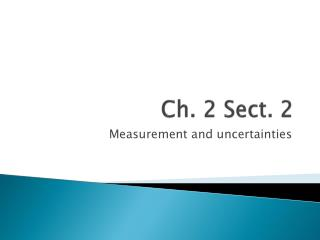DownloadDownload PresentationCh. 2 Sect. 2

# Ch. 2 Sect. 2

Download Presentation## Ch. 2 Sect. 2

- - - - - - - - - - - - - - - - - - - - - - - - - - - E N D - - - - - - - - - - - - - - - - - - - - - - - - - - -
##### Presentation Transcript

1. Ch. 2 Sect. 2 Measurement and uncertainties

2. Precision and Accuracy • Precision • Describes degree of exactness of a measurement • Which is more precise • Measurement A = 18.8 [+/-] 0.3 cm • Measurement B = 19.0 [+/-] 0.2 cm • Measurement C = 18.3 [+/-] 0.1 cm • Measurement C is more precise

3. Precision and Accuracy • Accuracy • Describes how well results of an experiment agree with standard value • If block from previous data was 19.0 cm, which measurement was most/least accurate? • Measurement B was most accurate • Measurement C was less accurate

4. Techniques of taking measurements • Make sure that your angle when taking a measurement is correct • Volume of a liquid  • Length on a meter-stick  • Parallax • Apparent shift in position of an object when veiwed at different angles

5. Significant Digits/Figures • Valid digits in a measurement

6. Measurement • Object A • Ruler 1- 6.80 cm • The “0” is the estimate or uncertain digit • There are 3 sig figs in this measurement • Ruler 2 – 6.8 cm • The “8” is your uncertain digit • There are only 2 sig figs • Which is more precise? • Ruler 1

7. Which Zero’s are significant? • If you answered measurement “0.0680 m” you would still only have 3 sig figs • First two zeros only locate the decimal (place holders) and aren’t significant • The last zero is the estimated digit • 186 000 m • 6 is the estimated digit while 0’s locate decimal • 3 sig figs • 186.000 km?? • 6 sig figs

8. Rules for Sig Figs • 1) Non zero digits are always significant • 2) All final zeros after the decimal point are significant • 3) Zeros between two other sig digits are significant • 4) Zeros that are used as place holders are not significant

9. Practice Problems • 2804 m • 2.84 km • 0.0029 m • 0.003068 m • 4.6 x 105 m • 4.06 x 10-5 m • 75.00 m • 0.007060 kg • 1.0080 x 107 km

10. Practice Problems • 2804 m  4 • 2.84 km  3 • 0.0029 m  2 • 0.003068 m  4 • 4.6 x 105 m  2 • 4.06 x 10-5 m  3 • 75.00 m  4 • 0.007060 kg  4 • 1.0080 x 107 km  5

11. Add and Subtract with sig figs • Important to remember that the result can never be more precise than the least precise measurement • When (+) or (-), first perform the operation then round to the least precise value • 24.686 m • 2.343 m • +3.21 m • =30.239  30.24 m

12. Multiply or divide • After (x) or (/) calculation, note the factor with least number of sig figs and round product or quotient to that number of digits • 3.22 cm x 2.1 cm • = 6.762  6.8 cm2